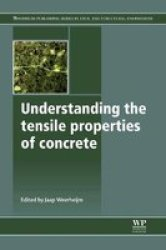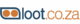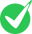## Understanding The Tensile Properties Of Concrete

From R3 534.00 at 1 ShopsWrite a Review

# Understanding The Tensile Properties Of Concrete

The response of concrete under tensile loading is crucial for most applications because concrete is much weaker in tension than in compression. Understanding the response mechanisms of concrete under tensile conditions is therefore key to understanding and using concrete in structural applications. ...
More details
From R3 534.00 at 1 Shop
0 Reviews

### All offers for Understanding The Tensile Properties Of ConcreteThe response of concrete under tensile loading is crucial for most applications because concrete is much weaker in tension than in compression. Understanding the response mechanisms of concrete under tensile conditions is therefore key to understanding and using concrete in structural applications. Understanding the tensile properties of concrete summarises key recent research in this important subject. After an introduction to concrete, the book is divided into two parts: part one on static response and part two on dynamic response. Part one starts with a summary chapter on the most important parameters that affect the tensile response of concrete. Chapters show how multi scale modelling is used to relate concrete composition to tensile properties. Part two focuses on dynamic response and starts with an introduction to the different regimes of dynamic loading, ranging from the low frequency loading by wind or earthquakes up to the extreme dynamic conditions due to explosions and ballistic impacts. Following chapters review dynamic testing techniques and devices that deal with the various regimes of dynamic loading. Later chapters highlight the dynamic behaviour of concrete from different viewpoints, and the book ends with a chapter on practical examples of how detailed knowledge on tensile properties is used by engineers in structural applications. Drawing on the work of some of the leading experts in the field, Understanding the tensile properties of concrete is a valuable reference for civil and structural engineers as well as those researching this important material. * Summarises key recent research in the areas of understanding the response mechanisms of concrete under tensile conditions* Provides a summary of the most important parameters that affect the tensile response of concrete and shows how multi scale modeling is used to relate concrete composition to tensile properties* Highlights the dynamic behaviour of concrete from different viewpoints and provides practical examples of how detailed knowledge on tensile properties is used by engineers in structural applications

#### All offers for Understanding The Tensile Properties Of ConcreteIncorrect offer? R0.00 R3 534.00
The response of concrete under tensile loading is crucial for most applications because concrete is much weaker in tension than in compression. Understanding the response mechanisms of concrete under tensile conditions is therefore key to understanding and using concrete in structural applications. Understanding the tensile properties of concrete summarises key recent research in this important subject. After an introduction to concrete, the book is divided into two parts: part one on static response and part two on dynamic response. Part one starts with a summary chapter on the most important parameters that affect the tensile response of concrete. Chapters show how multi scale modelling is used to relate concrete composition to tensile properties. Part two focuses on dynamic response and starts with an introduction to the different regimes of dynamic loading, ranging from the low frequency loading by wind or earthquakes up to the extreme dynamic conditions due to explosions and ballistic impacts. Following chapters review dynamic testing techniques and devices that deal with the various regimes of dynamic loading. Later chapters highlight the dynamic behaviour of concrete from different viewpoints, and the book ends with a chapter on practical examples of how detailed knowledge on tensile properties is used by engineers in structural applications. Drawing on the work of some of the leading experts in the field, Understanding the tensile properties of concrete is a valuable reference for civil and structural engineers as well as those researching this important material. * Summarises key recent research in the areas of understanding the response mechanisms of concrete under tensile conditions* Provides a summary of the most important parameters that affect the tensile response of concrete and shows how multi scale modeling is used to relate concrete composition to tensile properties* Highlights the dynamic behaviour of concrete from different viewpoints and provides practical examples of how detailed knowledge on tensile properties is used by engineers in structural applications##### 0 User Reviews

The response of concrete under tensile loading is crucial for most applications because concrete is much weaker in tension than in compression. Understanding the response mechanisms of concrete under tensile conditions is therefore key to understanding and using concrete in structural applications. Understanding the tensile properties of concrete summarises key recent research in this important subject. After an introduction to concrete, the book is divided into two parts: part one on static response and part two on dynamic response. Part one starts with a summary chapter on the most important parameters that affect the tensile response of concrete. Chapters show how multi scale modelling is used to relate concrete composition to tensile properties. Part two focuses on dynamic response and starts with an introduction to the different regimes of dynamic loading, ranging from the low frequency loading by wind or earthquakes up to the extreme dynamic conditions due to explosions and ballistic impacts. Following chapters review dynamic testing techniques and devices that deal with the various regimes of dynamic loading. Later chapters highlight the dynamic behaviour of concrete from different viewpoints, and the book ends with a chapter on practical examples of how detailed knowledge on tensile properties is used by engineers in structural applications. Drawing on the work of some of the leading experts in the field, Understanding the tensile properties of concrete is a valuable reference for civil and structural engineers as well as those researching this important material. * Summarises key recent research in the areas of understanding the response mechanisms of concrete under tensile conditions* Provides a summary of the most important parameters that affect the tensile response of concrete and shows how multi scale modeling is used to relate concrete composition to tensile properties* Highlights the dynamic behaviour of concrete from different viewpoints and provides practical examples of how detailed knowledge on tensile properties is used by engineers in structural applications#### 0 User Reviews